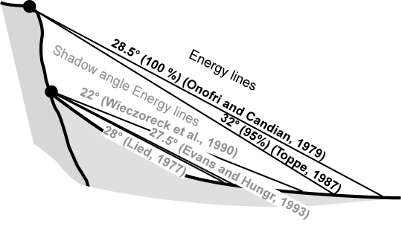## NAME

r.droka - Calculates run-out distance of a falling rock mass

## KEYWORDS

rock mass, rockfall

## SYNOPSIS

r.droka
r.droka --help
r.droka dem=string start=string ang=float red=float m=float num=integer prefix=name [n=integer] [--overwrite] [--help] [--verbose] [--quiet] [--ui]

### Flags:

--overwrite
Allow output files to overwrite existing files
--help
Print usage summary
--verbose
Verbose module output
--quiet
Quiet module output
--ui
Force launching GUI dialog

### Parameters:

dem=string [required]
Digital Elevation Model
start=string [required]
Name of starting points map
ang=float [required]
red=float [required]
Reduction value
Options: 0-1
Default: 0.9
m=float [required]
Value of rock mass (Kg)
num=integer [required]
Number of boulders (>=1)
prefix=name [required]
Prefix for output raster maps
n=integer
Buffer distance (meters)

## DESCRIPTION

The intersection between the topographic profile and the line starting from the point of detachment with a certain angle to the horizontal line defines the point of maximum propagation (Evans and Hungr, 1993; Jaboyedoff and Labiouse, 2003). The angle of inclination and the line of propagation are defined as zenith angle and shadow zone. This script defines rockfall zones from a digital elevation model (DEM) and vector layer containing starting point or points.Energy line used for the cone method from the top or the bottom of a cliff (shadow angle), according to various authors (Image from Jaboyedoff and Labiouse, 2003)

## INPUT

Digital Elevation Model = name

Name of elevation raster map

Starting points = name

Name of vector map containing rockfall source area. It can be one point or more.

Angle of inclination that defines the propagation zone. It's calculated from an horizontal plane starting from source point: highest values determine a lowest propagation.

Reduction value = double

Reduction moltiplicator for velocity. Default value is 0.9

Number of boulders = integer

For each starting point can be generated other random points used in the computations. By default points are located in a radius from the starting point equal to: (cell size * number of boulders)/2. Insert 1 for use only the original starting point or points. Note that a high number of boulders can make excessively long the calculation times.

Rock mass = double

Value of rock mass (Kg)

Output prefix = string

Name of prefix used for output raster maps

Buffer distance = integer

This value define the radius for randomly generatin boulders starting from starting point. It's in the option dialog window.

## OUTPUT

r.droka generates 5 raster maps and a vector map. The names of all output maps start with the prefix defined by the parameter prefix followed by name of map

• prefix_propagation: raster map rapresenting the area of propagation. If are used multiple source points, each pixel show the percentage of passage (%)
• prefix_vel_max: maximum rock-fall translational velocities (m/sec)
• prefix_vel_med: medium rock-fall translational velocities (m/sec)
• prefix_vel_max: medium kinetic energy (kJ)
• prefix_en_max: maximum kinetic energy (kJ)
• prefix_starting: vector map of starting points

## REFERENCES

FILIPELLO A., GIULIANI A., MANDRONE G. (2010) - Rock Slopes Failure Susceptibility Analysis: From Remote Sensing Measurements to Geographic Information System Raster Modules. American Journal of Environmental Sciences 6 (6): 489-494, 2010 ISSN 1553-345X 2010 Science Publications.

JABOYEDOFF M., LABIOUSE V. (2003) - Preliminary assessment of rockfall hazard based on GIS Data. Proceeding of the 10th ISRM Congress, South African Inst. Min. Met., Johannesburg, pp: 575-578

EVANS, S.G., HUNGR O. (1993). The assessment of rock fall hazard at the base of the talus slope. Can. Geotech. J., 30: 620-636. DOI: 10.1139/t93-054